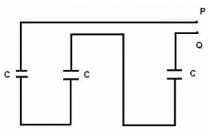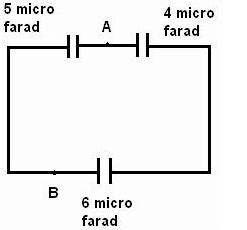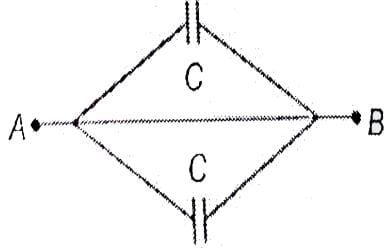NEET  >  Test: Combination of Capacitors

# Test: Combination of Capacitors

Test Description

## 10 Questions MCQ Test Physics Class 12 | Test: Combination of Capacitors

Test: Combination of Capacitors for NEET 2023 is part of Physics Class 12 preparation. The Test: Combination of Capacitors questions and answers have been prepared according to the NEET exam syllabus.The Test: Combination of Capacitors MCQs are made for NEET 2023 Exam. Find important definitions, questions, notes, meanings, examples, exercises, MCQs and online tests for Test: Combination of Capacitors below.
Solutions of Test: Combination of Capacitors questions in English are available as part of our Physics Class 12 for NEET & Test: Combination of Capacitors solutions in Hindi for Physics Class 12 course. Download more important topics, notes, lectures and mock test series for NEET Exam by signing up for free. Attempt Test: Combination of Capacitors | 10 questions in 10 minutes | Mock test for NEET preparation | Free important questions MCQ to study Physics Class 12 for NEET Exam | Download free PDF with solutions
 1 Crore+ students have signed up on EduRev. Have you?
Test: Combination of Capacitors - Question 1

### Here is a combination of three identical capacitors. If resultant capacitance is 1 μƒ, calculate capacitance of each capacitor.Detailed Solution for Test: Combination of Capacitors - Question 1

Combination of Capacitors in Series
C(effective) = C/3 = 1

Test: Combination of Capacitors - Question 2

### If the potential difference of a 6µF capacitor is changed from 10V to 20V, the increase in energy stored will be

Detailed Solution for Test: Combination of Capacitors - Question 2

Definition

Test: Combination of Capacitors - Question 3

### If two spheres of different radii have equal charge, then the potential will be

Detailed Solution for Test: Combination of Capacitors - Question 3

When equal charges are given to two spheres of different radii, the potential will be more or the smaller sphere as per the equation, Potential = Charge / Radius.
Since potential is inversely proportional to radius, the smaller radius will have higher potential and vice versa.

Test: Combination of Capacitors - Question 4

The capacitor preferred for high-frequency circuit is

Detailed Solution for Test: Combination of Capacitors - Question 4

Mica capacitor. Mica capacitors have low resistive and inductive components associated with it. Hence, they have high Q factor and because of high Q factor their characteristics are mostly frequency independent, which allows this capacitor to work at high frequency.

Test: Combination of Capacitors - Question 5

Three different capacitors are connected in series, then:

Detailed Solution for Test: Combination of Capacitors - Question 5

C = Q/V
series connection splits the battery potential, hence....
V = V1 + V2
V1 = Q/C1 & V2 = Q/C2
Therefore, both have equal charge

Test: Combination of Capacitors - Question 6

Calculate the equivalent capacitance for the following combination between points A and BDetailed Solution for Test: Combination of Capacitors - Question 6

4μf and 6μf are in series (right side of AB) so 6x(4/6)+4=24/10μf
And now 5 μf and the resulting of above two are in parallel (as on different sides of AB) so 5+(24/10) =50+(24/10)=74/10=37/5μf

Test: Combination of Capacitors - Question 7

There are three capacitors with equal capacitance. In series combination, they have a net capacitance of Cand in parallel combination, a net capacitance of C2.What will be the value of C1 C2?​

Detailed Solution for Test: Combination of Capacitors - Question 7

For series combination,
1/C1=(1/C)+(1/C)+(1/C) [ Since all capacitors have equal capacitances]=3/C Or
C1=C/3−−−(i)
For parallel combination,
C2=C+C+C=3C−−−(ii)
Now,C1/C2=(C/3)/3C=(C/3)×(1/3C)=1/9

Test: Combination of Capacitors - Question 8

Two capacitors of 20 μƒ and 30 μƒ are connected in series to a battery of 40V. Calculate charge on each capacitor.​

Detailed Solution for Test: Combination of Capacitors - Question 8

C1= 20×10µf

and C2= 30×10µf

in series Ceq = C1C2/(C1+C2)

Ceq = 20×10^(-6)×30×10^(-6)/20×10^(-6)+30^×10(-6)

Ceq= 12×10^(-6)f

As we know that Q = CV

Putting the values of C and V= 40V, we get

Q = (12 * 10^-6) * 40

= 480µC

Test: Combination of Capacitors - Question 9

Two capacitors of equal capacity are first connected in parallel and then in series. The ratio of the total capacities in the two cases will be

Detailed Solution for Test: Combination of Capacitors - Question 9

Third capacitor is short-circuited as its both ends are connected to B. Equivalent circuit isCeq=C+C=2C

Test: Combination of Capacitors - Question 10

When two capacitors C1 and C2 are connected in series and parallel, their equivalent capacitances comes out to be 3μƒ and 16μƒ respectively. Calculate values of C1 and C2.

Detailed Solution for Test: Combination of Capacitors - Question 10

Let Cp be the equivalent capacitance of parallel
Cp=C1+C2
16= C1+C2……..(1)
Let Cs be the equivalent of capacitance in series
1/Cs=(1/C1) + (1/C2)
Or, Cs=C1C2/C1+C2
Or,3=C1C2/16
Or,C2C1=48
C2=48/C1……..(ii)
16=C1+48/C1
(C12-16C1+48)=0
(C1-4)(C1-12)=0
C1=4 ; C1=12
If,
C1=4
C2=12
If,
C2=4
C1=12
So, the answer is, Either 12μf or4μf

## Physics Class 12

157 videos|452 docs|213 tests
 Use Code STAYHOME200 and get INR 200 additional OFF Use Coupon Code
Information about Test: Combination of Capacitors Page
In this test you can find the Exam questions for Test: Combination of Capacitors solved & explained in the simplest way possible. Besides giving Questions and answers for Test: Combination of Capacitors, EduRev gives you an ample number of Online tests for practice

## Physics Class 12

157 videos|452 docs|213 tests

### How to Prepare for NEET

Read our guide to prepare for NEET which is created by Toppers & the best Teachers